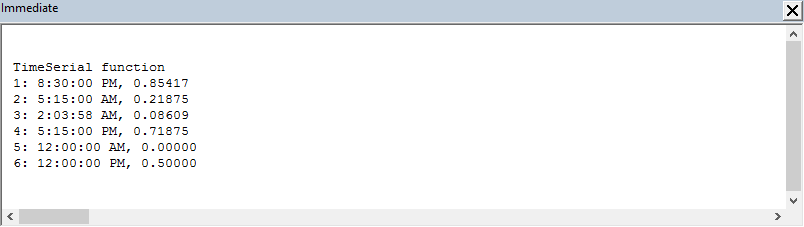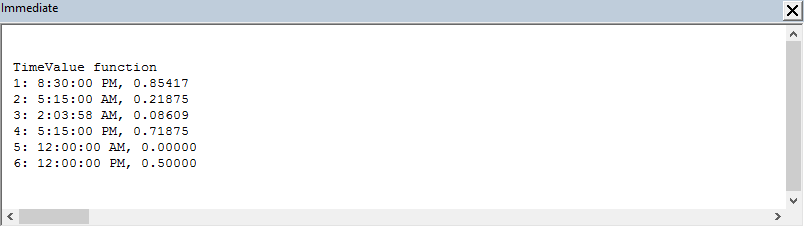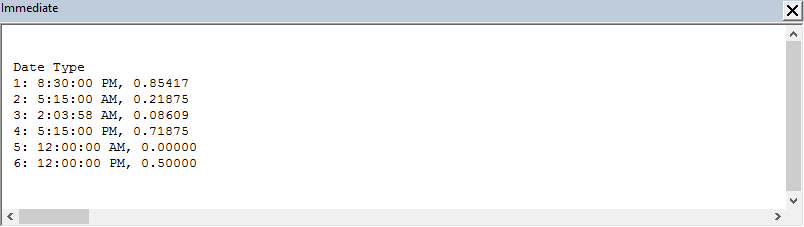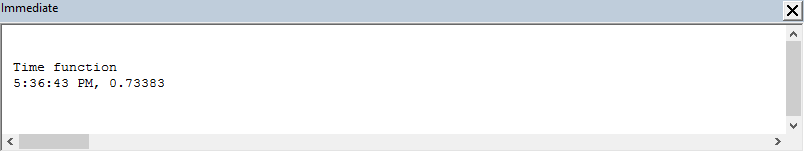# VBA time concepts

VBA concepts in this module:

• TimeSerial function
• TimeValue function
• VBA Date type
• Time function

The same time set is used in all examples.

VBA times range from #00:00:00#, 12 midnight (#00:00:00# automatically converts to #12:00:00 AM#), to #23:59:59#, one minute to midnight. The smallest time increment is #00:00:01#, 1 second.TimeSerial(20,30,00) returns 8:30:00 PM.TimeValue("20:30") returns 8:30:00 PM,t = #20:30# assigns #8:30:00 PM# to t, and returns 8:30:00 PM

## 2.1 TimeSerial function

VBA function properties Description
TimeSerial(hour, minute, second)Returns a Date value representing specified Integer values for hour, minute, and second - for day zero
CDbl(expression)Converts a text string or numeric value to a double precision floating point number

Six sample times are assigned to the TimeArray (TA) in code 1. For example, 20:30:00 (hh:mm:ss) is stored in the three elements of row 1 (code 1, line 6). Using an array enables the use of a For...Next loop to write to the Immediate Window.

Code 1: TimeSerial function
' 1. === TimeSerialFunction
Sub TimeSerialFunction()
Dim TA(1 To 6, 1 To 3) As Integer   'TimeArray
Dim i As Integer

TA(1, 1) = 20:   TA(1, 2) = 30:   TA(1, 3) = 0	' 20:30:00
TA(2, 1) = 5:    TA(2, 2) = 15:   TA(1, 3) = 0
TA(3, 1) = 2:   TA(3, 2) = 3:   TA(3, 3) = 58
TA(4, 1) = Hour("5 PM")
TA(4, 2) = 15
TA(4, 3) = 0
TA(5, 1) = 0:    TA(5, 2) = 0:    TA(5, 3) = 0
TA(6, 1) = 12:   TA(6, 2) = 0:    TA(6, 3) = 0	' 12:00:00

Debug.Print vbNewLine
Debug.Print "TimeSerial function"
For i = 1 To UBound(TA)
Debug.Print i & ": " & TimeSerial(TA(i, 1), TA(i, 2), TA(i, 3)) _
& ", " & Format(CDbl(TimeSerial(TA(i, 1), TA(i, 2), TA(i, 3))), "#0.00000")
Next i

End Sub


The TimeSerial function is used in line 18 to return the Date value as shown in the Immediate Window (figure 1).Fig 1: Immediate Window - showing the output of Code 1

## 2.2 TimeValue function

VBA function properties Description
TimeValue(time)Takes Time as a String, and returns a Date value representing the time for day zero.

The colon (:) date operator is common to VBA and Excel. TimeValue is used in code 2, line 18 to return the time values shown in figure 2.

Code 2: TimeValue (Code)
' 2. === TimeValueFunction
Sub TimeValueFunction()
Dim TA(1 To 6) As String    'TimeArray
Dim i As Integer

TA(1) = "20:30"
TA(2) = "5:15"
TA(3) = "2:03:58"
TA(4) = "5:15 PM"
TA(5) = "0:0"
TA(6) = "12:00"

Debug.Print vbNewLine
Debug.Print "TimeValue function"
For i = 1 To UBound(TA)
Debug.Print i & ": " & TimeValue(TA(i)) & ", " & Format(CDbl(TimeValue(TA(i))), "#0.00000")
Next i

End SubFig 2: Immediate Window - showing the output of code 2

## 2.3 Date type

In this example the TimeArray (TA) is populated with sample times as VBA Date type. The Visual Basic Editor automatically converts times such as 8:30 PM, entered as #20:30#, to #8:30:00 PM# on completion of line (see code 3, line 6).

Code 3: Module ThisWorkbook (Code)
' 3. === DateType
Sub DateType()
Dim TA(1 To 6) As Date    'TimeArray
Dim i As Integer

TA(1) = #8:30:00 PM#    ' The VBE automatically converts #20:30# to #8:30:00 PM#
TA(2) = #5:15:00 AM#
TA(3) = #2:03:58 AM#
TA(4) = #5:15:00 PM#
TA(5) = #12:00:00 AM#
TA(6) = #12:00:00 PM#

Debug.Print vbNewLine
Debug.Print "Date Type"
For i = 1 To UBound(TA)
Debug.Print i & ": " & (TA(i)) & ", " & Format(CDbl((TA(i))), "#0.00000")
Next i

End SubFig 3: Immediate Window - showing the output of code 3

## 3.4 Time function

VBA function properties Description
Time()Returns the current system time. (No arguments)

Code 4: Module1  (Code)
' 4. === TimeFunction
Sub TimeFunction()

Debug.Print vbNewLine
Debug.Print "Time function"
Debug.Print Time & ", " & Format(CDbl(Time), "#0.00000")

End Sub


The Time function takes no arguments and can be entered as Time, or Time().Fig 4: Immediate Window - showing the output of code 4.

The VBE derives its default time format from the Windows Regional Settings - Long Time hh:mm:ss tt. This explains the converted values in the TA array (code 3). The Format function, Format(expression, "C") is an equivalent to the Windows Long Time default.The default date format is the same in VBA (code 3 above) and the time values returned in the Excel formula bar. Each is based on the Windows Regional Date and Time formats - Long Time